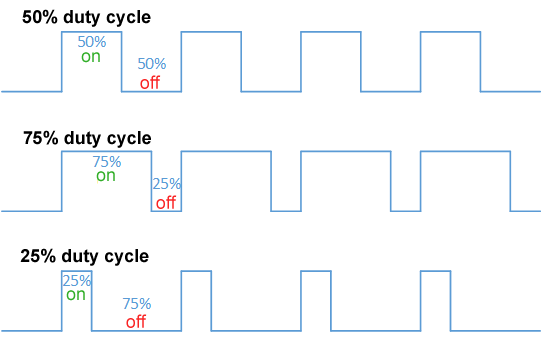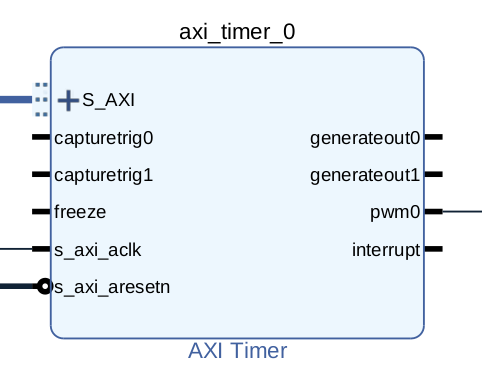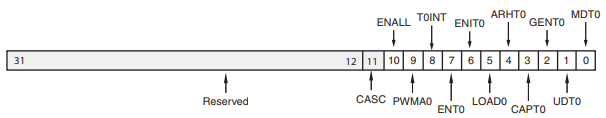## Introduction

PYNQ is a perfect framework for beginners and advanced users of FPGA,  it provides a great base bitstream that manages some peripherals on the board without any effort. However, if you need custom IPs for your application (and also for custom pinout), you need to know how to create a specific bitstream using the Xilinx IP Cores, which are quite documented but not often ready to use. In this article, we can show you how to implement a design that can output multiple PWM signals on the PMOD of a PYNQ Z2 board. In the next episode, we will show you how to control multiple stepper motors.

## What is PWM signal

Pulse width modulation (PWM) is a modulation technique that generates variable-width pulses to represent the amplitude of an analog input signal.

We are interested in two parameters: signal frequency (or period), and the signal duty cycle:

• The Period is the time that the signal takes to be low and high
• The duty cycle is the ratio of the high time to the period of the signal.## IP core for PWM: the Axi Timer

The only IP core that provides Xilinx that can generate a PWM signal is the Axi Timer. Axi Timer is controlled by a S_AXI interface and it get outs two kinds of outputs:

• a timer output, for generating a simple clock timer
• a PWM, for generating a PWM signal

So, according to that, we will create a design that generates a PWM signal connected to a specific PIN of the PYNQ Z2.The Vivado flow is the usual flow:

• add the Zynq processing system
• place an Axi interconnect, with Master Axi as many as you need for connecting Axi Timer
• add some Axi Timer (as many as you need for PWM signals)
• create pinout interfaces with the right constraints according to the PMOD pinout,)
• connect all together

For instance, this is a possible design for the purpose:pretty cool, uhm?

In our design, we have defined this constraint file (we need 8 PWM signals, so we have connect all of the PMODB pins):

``````set_property -dict {PACKAGE_PIN Y14 IOSTANDARD LVCMOS33} [get_ports {motor1}]
set_property -dict {PACKAGE_PIN W14 IOSTANDARD LVCMOS33} [get_ports {motor2}]
set_property -dict {PACKAGE_PIN T10 IOSTANDARD LVCMOS33} [get_ports {motor3}]
set_property -dict {PACKAGE_PIN T11 IOSTANDARD LVCMOS33} [get_ports {motor4}]
set_property -dict {PACKAGE_PIN W16 IOSTANDARD LVCMOS33} [get_ports {motor5}]
set_property -dict {PACKAGE_PIN V16 IOSTANDARD LVCMOS33} [get_ports {motor6}]
set_property -dict {PACKAGE_PIN W13 IOSTANDARD LVCMOS33} [get_ports {motor7}]
set_property -dict {PACKAGE_PIN V12 IOSTANDARD LVCMOS33} [get_ports {motor8}]
``````

After that, generate the bitstream. Okay, everyone, here comes the fun part.

## Pynq code for PWM generation

So, now we have the bitstream. How we can use it? You must read the documentation of the Axi Timer😋
The Axi Timer documentation is here: https://www.xilinx.com/support/documentation/ip_documentation/axi_timer/v2_0/pg079-axi-timer.pdf

As with every peripheral, you need to control the control/status register shown below:So, we need to write on the specific bits of this register the value in order to activate the PWM signal generator.

In particular, we need this configuration:

• The PWMA0 bit in TCSR0 and PWMB0 bit in TCSR1 must be set to 1 to enable PWM mode
• The GenerateOut signals must be enabled in the TCSR (bit GENT set to 1). The PWM0 signal is generated from the GenerateOut signals of Timer 0 and Timer 1, so these signals must be enabled in both timer/counters;
• The counter UDT can be set to count up or down (we prefer to set to 1);
•  set Autoreload register ARHT0 to 1;
• enable timer setting ENT0 to 1.

After that, we can set the period and the duty cycle of the generated PWM signal.

Ok, now we are ready to control the PWM! Here is some PYNQ code for PWM generation (on single Axi Timer)

``````
from pynq import Overlay

# utility functions for bit manipulation
def set_bit(value, bit):
return value | (1 << bit)

def clear_bit(value, bit):
return value & ~(1 << bit)

def get_bit(value, bit):
return (value >> bit) & 1

ol = Overlay("pwm_stepper_motor.bit")
motor1 = ol.axi_timer_0

# extract register addresses (will be the same for every Axi Timer)
TCSR0 = ol.ip_dict['axi_timer_0']['registers']['TCSR0']
TCSR1 = ol.ip_dict['axi_timer_0']['registers']['TCSR1']
TCSR0_register = TCSR0['fields'] # bit_offset for address
TCSR1_register = TCSR1['fields']
TLR0 = ol.ip_dict['axi_timer_0']['registers']['TLR0']
TLR1 = ol.ip_dict['axi_timer_0']['registers']['TLR1']

# create the configuration values for the control register
temp_val_0 = 0
temp_val_1 = 0

# The PWMA0 bit in TCSR0 and PWMB0 bit in TCSR1 must be set to 1 to enable PWM mode.
temp_val_0 = set_bit(temp_val_0, TCSR0_register['PWMA0']['bit_offset'])
temp_val_1 = set_bit(temp_val_1, TCSR1_register['PWMA1']['bit_offset'])

# The GenerateOut signals must be enabled in the TCSR (bit GENT set to 1). The PWM0
# signal is generated from the GenerateOut signals of Timer 0 and Timer 1, so these
# signals must be enabled in both timer/counters
temp_val_0 = set_bit(temp_val_0, TCSR0_register['GENT0']['bit_offset'])
temp_val_1 = set_bit(temp_val_1, TCSR1_register['GENT1']['bit_offset'])

# The counter can be set to count up or down. UDT
temp_val_0 = set_bit(temp_val_0, TCSR0_register['UDT0']['bit_offset'])
temp_val_1 = set_bit(temp_val_1, TCSR1_register['UDT1']['bit_offset'])

# set Autoreload (ARHT0 = 1)
temp_val_0 = set_bit(temp_val_0, TCSR0_register['ARHT0']['bit_offset'])
temp_val_1 = set_bit(temp_val_1, TCSR1_register['ARHT1']['bit_offset'])

# enable timer (ENT0 = 1)
temp_val_0 = set_bit(temp_val_0, TCSR0_register['ENT0']['bit_offset'])
temp_val_1 = set_bit(temp_val_1, TCSR1_register['ENT1']['bit_offset'])

# here you must see "0b1010010110" twice
print(bin(temp_val_0))
print(bin(temp_val_1))

# Now we can generate the PWM!
_period_ = 20000 # 50Hz, 20ms
_pulse_ = 50 # 50% duty cycle
period = int((_period_ & 0x0ffff) *100);
pulse = int((_pulse_ & 0x07f)*period/100);
print("period 20ms", period)
print("pulse 50%", pulse)

``````

If you connect to pin 2 of the PMOD B with an oscilloscope, you will see a square signal!

If you want to change the period of the PWM with a graphical interface, you can use this Jupyter Notebook snippet:

``````
from ipywidgets import interact, interactive, HBox, VBox
import ipywidgets as widgets

def clicked(P=None, Torque=None):

_period_ = P
_pulse_ = 50 # 50% duty cycle
period = int((_period_ & 0x0ffff) *100);
pulse = int((_pulse_ & 0x07f)*period/100);
print("period 20ms", period)
print("pulse 50%", pulse)

w = interactive(clicked,
P=widgets.IntSlider(min=4000, max=30000, step=1, value=4000),
Torque=widgets.IntSlider(min=-400, max=400, step=1, value=0))
VBox([w.children,
])
``````

## Conclusion

Now we have the possibility to generate PWM signals with a custom bitstream for PYNQ. We also see that exists the Axi Timer provided by Xilinx that allows you to create this particular signal. So, what are the next steps? We will show you how to control a stepper motor with a PYNQ Z2 board.